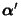## Principal Curve

A curveon a Regular Surfaceis a principal curve Iff the velocityalways points in a Principal Direction, i.e.,whereis the Shape Operator andis a Principal Curvature. If a Surface of Revolution generated by a plane curve is a Regular Surface, then the Meridians and Parallels are principal curves.

References

Gray, A. Principal Curves'' and The Differential Equation for the Principal Curves.'' §18.1 and 21.1 in Modern Differential Geometry of Curves and Surfaces. Boca Raton, FL: CRC Press, pp. 410-413, 1993.